# Python Program to Check if a Number is Odd or Even

In this tutorial you will learn about the Python Program to Check if a Number is Odd or Even and its application with practical example.

In this tutorial, we will learn to create a Python Program to Check if a Number is Odd or Even using python Programming.

## Prerequisites

Before starting with this tutorial we assume that you are best aware of the following Python programming topics:

• Python Operator.
• Basic Input and Output
• Basic Python  programming.
• Python if-else statements.
• Python Loop.

## What is Even Number?

A Number is a Even number that can be divided by two is Even number and end  usually with 0, 2, 4, 6, 8.

## What is Odd Numbers?

Odd Number is just reverse of Even Number they cannot be divisible by 2 and usually ends with 1,3,5,7,9.

## Python Program to Check if a Number is Odd or Even

In our program below we will check given number is Even or Odd .First we would declared and initialized the required variables. Next, we would prompt user to input the value. Later we will find entered value is Even or Odd.

## Output

Even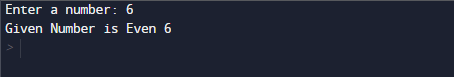Odd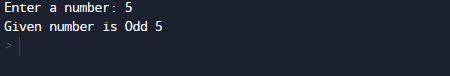In our program, we have first declared and initialized a set variables required in the program.

• number =taking year value from user.

First of all  we declare variable and take a value from user to check whether a entered number is Even or Odd.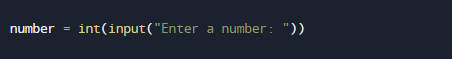After taking values from user we will check that if a number is completely divisible by 2 then the given number is Even.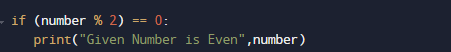If the given number is not divisible by 2 that means the given number is Odd.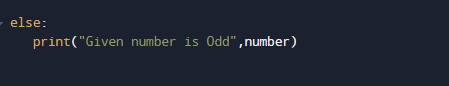So a number is even if it is completely divisible by 2 (number % 2== 0) gives remainder zero( 0 ). When the given number is divided by 2,here we will use the modulus operator % to find the remainder. If the remainder is zero, the number is Even if not number is Odd.

In this tutorial we have learn about the Python Program to Check if a Number is Odd or Even and its application with practical example. I hope you will like this tutorial.